Tamilnadu State Board New Syllabus Samacheer Kalvi 12th Maths Guide Pdf Chapter 4 Inverse Trigonometric Functions Ex 4.6 Textbook Questions and Answers, Notes.

## Tamilnadu Samacheer Kalvi 12th Maths Solutions Chapter 4 Inverse Trigonometric Functions Ex 4.6

Choose the most suitable answer from the given four alternatives:

Question 1.
The value of sin-1(cos x), 0 ≤ x ≤ π is
(a) π – x
(b) x – $$\frac {π}{2}$$
(c) $$\frac {π}{2}$$ – x
(d) x – π
Solution:
(c) $$\frac {π}{2}$$ – x
Hint:
sin-1(cos x) = sin-1(sin($$\frac {π}{2}$$ – x)) = $$\frac {π}{2}$$ – x

Question 2.
If sin-1 x + sin-1 y = $$\frac {2π}{3}$$; then cos-1 x + cos-1 y is equal to
(a) $$\frac {2π}{3}$$
(b) $$\frac {π}{3}$$
(c) $$\frac {π}{6}$$
(d) π
Solution:
(b) $$\frac {π}{3}$$
Hint:
sin-1x + cos-1x + cos-1y + sin-1y = $$\frac {π}{2}$$ + $$\frac {π}{2}$$ = π
$$\frac {2π}{3}$$ + cos-1x + cos-1y = π
cos-1x + cos-1y = π – $$\frac {2π}{3}$$ = $$\frac {3π-2π}{3}$$ = $$\frac {π}{3}$$Question 3.
sin-1$$\frac {3}{5}$$ – cos-1$$\frac {12}{13}$$ + sec-1$$\frac {5}{3}$$ – cosec-1$$\frac {13}{12}$$ is equal to
(a) 2π
(b) π
(c) 0
(d) tan-1$$\frac {12}{65}$$
Solution:
(c) 0
Hint: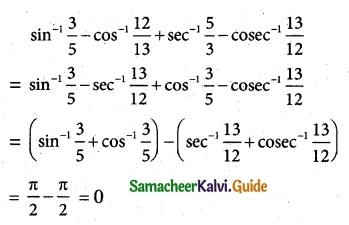Question 4.
If sin-1 x = 2sin-1 α has a solution, then
(a) |α| ≤ $$\frac {1}{√2}$$
(b) |α| ≥ $$\frac {1}{√2}$$
(c) |α| < $$\frac {1}{√2}$$
(d) |α| > $$\frac {1}{√2}$$
Solution:
(a) |α| ≤ $$\frac {1}{√2}$$
Hint:
If sin-1 x = 2sin-1 α has a solution then
–$$\frac {π}{2}$$ ≤ 2sin-1α ≤ $$\frac {π}{2}$$
–$$\frac {π}{4}$$ ≤ sin-1α ≤ $$\frac {π}{4}$$
sin($$\frac {-π}{4}$$) ≤ α ≤ sin$$\frac {π}{4}$$
–$$\frac {1}{√2}$$ ≤ α ≤ $$\frac {1}{√2}$$
-|α| ≤ $$\frac {1}{√2}$$Question 5.
sin-1(cos x) = $$\frac {π}{2}$$ – x is valid for
(a) -π ≤ x ≤ 0
(b) 0 ≤ x ≤ π
(c) –$$\frac {π}{2}$$ ≤ x ≤ $$\frac {π}{2}$$
(d) –$$\frac {π}{4}$$ ≤ x ≤ $$\frac {3π}{4}$$
Solution:
(b) 0 ≤ x ≤ π
Hint:
sin-1 (cosx) = $$\frac {π}{2}$$ – x is valid for
cos x = sin ($$\frac {π}{2}$$ – x)
cos x ∈ [0, π]
∴ 0 ≤ x ≤ π

Question 6.
If sin-1 x + sin-1 y + sin-1 z = $$\frac {3π}{2}$$, the value of show that x2017 + y2018 + z2019 – $$\frac {9}{x^{101}+y^{101}+z^{101}}$$ is
(a) 0
(b) 1
(c) 2
(d) 3
Solution:
(a) 0
Hint: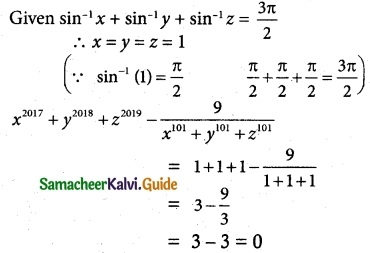Question 7.
If cot-1 x = $$\frac {2π}{5}$$ for some x ∈ R, the value of tan-1 x is
(a) –$$\frac {π}{10}$$
(b) $$\frac {π}{5}$$
(c) $$\frac {π}{10}$$
(d) –$$\frac {π}{5}$$
Solution:
(c) $$\frac {π}{10}$$
Hint:
tan-1 x + cos-1 $$\frac {π}{2}$$
tan-1x = $$\frac {π}{2}$$ – cot-1 x = $$\frac {π}{2}$$ – $$\frac {2π}{5}$$
= $$\frac {5π-4π}{10}$$ = $$\frac {π}{10}$$

Question 8.
The domain of the function defined by f(x) = sin-1 $$\sqrt {x-1}$$ is
(a) [1, 2]
(b) [-1, 1]
(c) [0, 1]
(d) [-1, 0]
Solution:
(a) [1, 2]
Hint:
f(x) = sin-1 $$\sqrt {x-1}$$
$$\sqrt {x-1}$$ ≥ 0
-1 ≤ $$\sqrt {x-1}$$ ≤ 1
∴ 0 ≤ $$\sqrt {x-1}$$ ≤ 1
0 ≤ x – 1 ≤ 1
1 ≤ x ≤ 2
x ∈ [1, 2]Question 9.
If x = $$\frac {1}{5}$$ the value of cos(cos-1x + 2sin-1x) is
(a) –$$\sqrt{\frac {24}{25}}$$
(b) $$\sqrt{\frac {24}{25}}$$
(c) $$\frac {1}{5}$$
(d) –$$\frac {1}{5}$$
Solution:
(d) –$$\frac {1}{5}$$
Hint:
cos[cos-1x + sin-1x + sin-1x] = cos($$\frac {π}{2}$$ + sin-1x)
= -sin(sin-1x)
[∵ cos(90+θ) = -sin θ]
= -x = –$$\frac {1}{5}$$

Question 10.
tan-1($$\frac {1}{4}$$) + tan-1($$\frac {2}{9}$$) is equal to
(a) $$\frac {1}{2}$$cos-1($$\frac {3}{5}$$)
(b) $$\frac {1}{2}$$sins-1($$\frac {3}{5}$$)
(c) $$\frac {1}{2}$$tan-1($$\frac {3}{5}$$)
(d) tan-1($$\frac {1}{2}$$)
Solution:
(d) tan-1($$\frac {1}{2}$$)
Hint: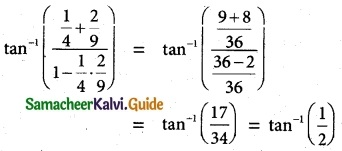Question 11.
If the function f(x) = sin-1(x² – 3), then x belongs to
(a) [-1, 1]
(b) [√2, 2]
(c) [-2, -√2]∪[√2, 2]
(d) [-2, -√2]
Solution:
(c) [-2, -√2]∪[√2, 2]
Hint:
-1 ≤ x² – 3 ≤ 1
-1 + 3 ≤ x² ≤ 1 + 3
⇒ 2 ≤ x² ≤ 4
±√2 ≤ x ≤ ± 2
[-2, -√2]∪[√2, 2]

Question 12.
If cot-1 2 and cot-1 3 are two angles of a triangle, then the third angle is
(a) $$\frac {π}{4}$$
(b) $$\frac {3π}{4}$$
(c) $$\frac {π}{6}$$
(d) $$\frac {π}{3}$$
Solution:
(b) $$\frac {3π}{4}$$
Hint:
A + B + C = π (triangle)
cot-1 2 + cot-1 3 + C = π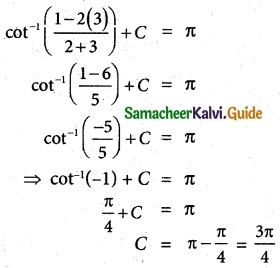Question 13.
sin-1(tan$$\frac {π}{4}$$) – sin-1($$\sqrt{\frac {3}{x}}$$) = $$\frac {π}{6}$$. Then x is root of the equation
(a) x² – x – 6 = 0
(b) x² – x – 12 = 0
(c) x² + x – 12 = 0
(d) x² + x – 6 = 0
Solution:
(b) x² – x – 12 = 0
Hint: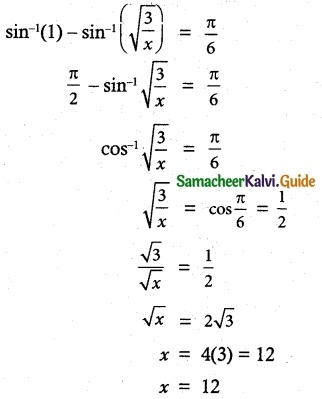Question 14.
sin-1(2 cos²x – 1) + cos-1(1 – 2 sin²x) =
(a) $$\frac {π}{2}$$
(b) $$\frac {π}{3}$$
(c) $$\frac {π}{4}$$
(d) $$\frac {π}{6}$$
Solution:
(a) $$\frac {π}{2}$$
Hint:
sin-1(2 cos² x – 1) + cos-1(1 – 2 sin²x)
= sin-1 (2 cos² x – 1) + cos-1 (1 – sin² x – sin² x)
= sin-1(2 cos² x – 1) + cos-1(cos² x – (1 – cos²x))
= sin-1(2 cos² x – 1) + cos-1(cos² x – 1 + cos²x)
= sin-1(2 cos² x – 1) + cos-1(2 cos² x – 1)
= $$\frac {π}{2}$$ [∵ sin-1 x + cos-1 x = $$\frac {π}{2}$$]Question 15.
If cot-1($$\sqrt {sinα}$$) + tan-1($$\sqrt {sinα}$$) = u, then cos 2u is equal to
(a) tan²α
(b) 0
(c) -1
(d) tan 2α
Solution:
(c) -1
Hint:
cot-1 x + tan-1 x = $$\frac {π}{2}$$
∴ u = $$\frac {π}{2}$$
cos 2u = cos 2($$\frac {π}{2}$$) = cos π = -1

Question 16.
If |x| ≤ 1, then 2 tan-1 x – sin-1$$\frac {2x}{1+x²}$$ is equal to
(a) tan-1x
(b) sin-1x
(c) 0
(d) π
Solution:
(c) 0
Hint:
sin-1$$\frac {2x}{1+x²}$$ = 2 tan-1x
∴ 2 tan-1 x – 2 tan-1 x = 0Question 17.
The equation tan-1 x – cot-1 x = tan-1($$\frac {1}{√3}$$) has
(a) no solution
(b) unique solution
(c) two solutions
(d) infinite number of solutions
Solution:
(b) unique solution
Hint:
tan-1 x – cot-1 x = tan-1($$\frac {1}{√3}$$) …….. (1)
tan-1 x – cot-1 x = $$\frac {π}{2}$$ ……… (2)
2 tan-1 x = $$\frac {π}{6}$$ + $$\frac {π}{2}$$ = $$\frac {2π}{3}$$
tan-1 x = $$\frac {π}{3}$$
x = √3 which is uniqe solution.

Question 18.
If sin-1 x + cot-1($$\frac {1}{2}$$) = $$\frac {π}{2}$$, then x is equal to
(a) $$\frac {1}{2}$$
(b) $$\frac {1}{√5}$$
(c) $$\frac {2}{√5}$$
(d) $$\frac {√3}{2}$$
Solution:
(b) $$\frac {1}{√5}$$
Hint: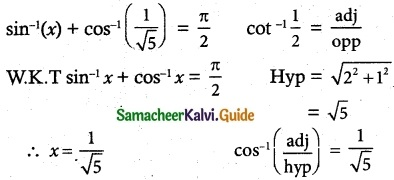Question 19.
If sin-1 $$\frac {x}{5}$$ + cosec-1$$\frac {5}{4}$$ = $$\frac {π}{2}$$, then the value of x is
(a) 4
(b) 5
(c) 2
(d) 3
Solution:
(d) 3
Hint: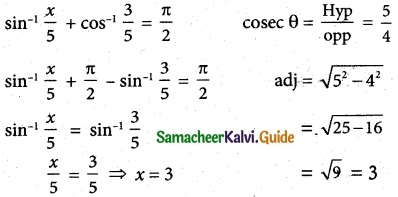Question 20.
sin(tan-1 x), |x| < 1 is equal to
(a) $$\frac {x}{\sqrt{1-x^2}}$$
(b) $$\frac {1}{\sqrt{1-x^2}}$$
(c) $$\frac {1}{\sqrt{1+x^2}}$$
(d) $$\frac {x}{\sqrt{1+x^2}}$$
Solution:
(d) $$\frac {x}{\sqrt{1+x^2}}$$
Hint:
tan a = x
W.K.T 1 + tan² a = sec² a
1 + x² = sec² a
sec a = $$\sqrt{1+x^2}$$
$$\frac {1}{cosa}$$ = $$\sqrt{1+x^2}$$
cos a= $$\frac {1}{\sqrt{1+x^2}}$$
sin a = $$\sqrt{1-cos^2a}$$ = $$\sqrt{1-\frac {1}{1+x^2}}$$
$$\sqrt{\frac{1+x^2 -1}{1+x^2}}$$ = $$\frac {x}{\sqrt{1+x^2}}$$